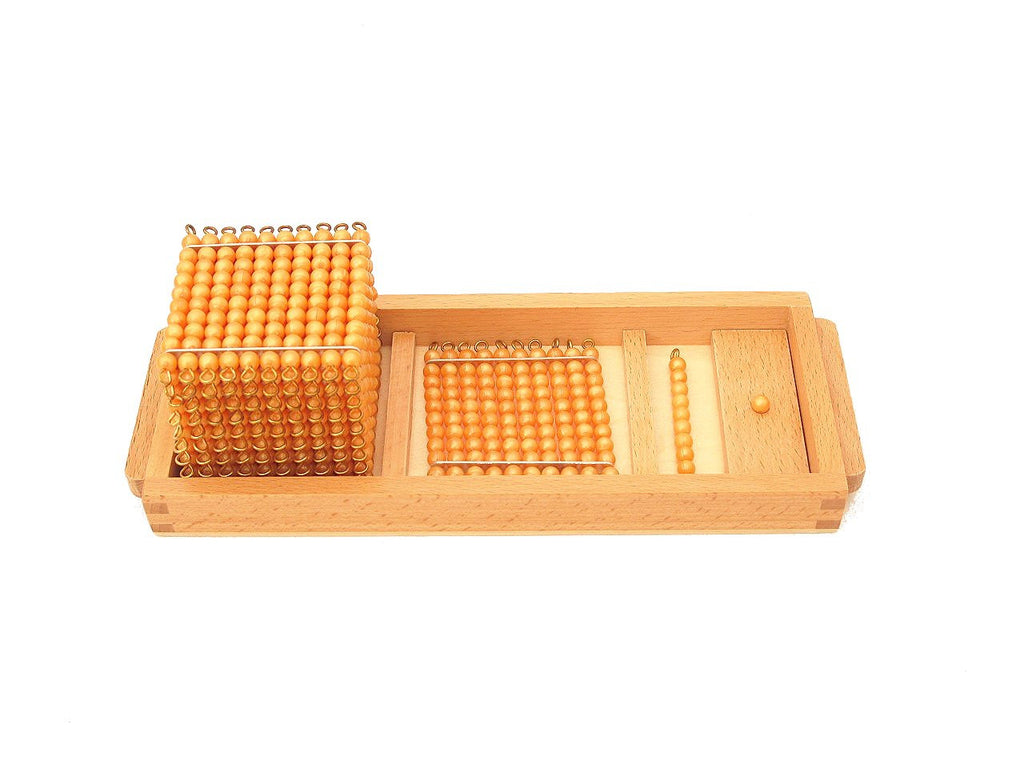### Introduction to Decimal Quantity

\$39.99 USD

Montessori Mathematics Material

The golden bead units, ten bars, hundred squares, and thousand cubes are used mainly in Montessori decimal system to demonstrate values of ones, tens, hundreds, and thousands; to associate beads to numbers; and to operate mathematical equations in addition, subtraction, multiplication and division.

What's Included:

• A wooden compartment tray
• one unit bead and a stand
• one bead bar of ten
• one hundred square
• one thousand cube

Sale

Unavailable

Sold Out